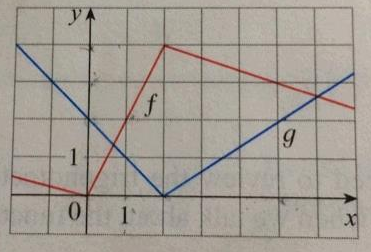# If f and g are the functions whose graphs are shown, let u(x) = f(x)g(x) and v(x) = f(x)/g(x)

If f and g are the functions whose graphs are shown, let
u(x)=f(x)g(x) and v(x)=f(x)/g(x).
(a) Find u′(1).
(b) Find v′(5).

||